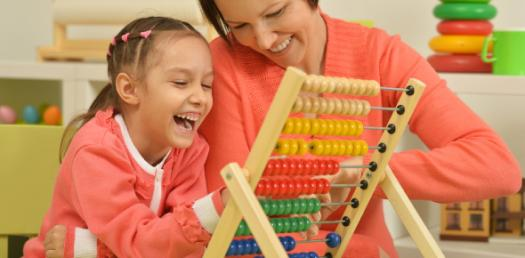# Math: Addition Trivia Quiz! Test Yourself

48 Questions | Total Attempts: 73SettingsAre you looking for a trivia quiz to test yourself on solving math problems on addition? You are inluck as the quiz below is perfect for that. Give it a try and be sure to rake as much time as you need before you choose the answer you see fit. All the best and keep a look out for other quizzes like it.

Related Topics
• 1.
9+8=
• 2.
5+6=
• 3.
8+9=
• 4.
7+6=
• 5.
6+9=
• 6.
9+4=
• 7.
4+8=
• 8.
5+4=
• 9.
8+4=
• 10.
7+7=
• 11.
8+9=
• 12.
9+8=
• 13.
6+6=
• 14.
5+8=
• 15.
7+6=
• 16.
3+4=
• 17.
8+8=
• 18.
8+9=
• 19.
7+8=
• 20.
6+6=
• 21.
5+3=
• 22.
7+9=
• 23.
8+8=
• 24.
4+8=
• 25.
0+8=